## February 19, 2018

### Cartesian Bicategories

#### Posted by John Baezguest post by Daniel Cicala and Jules Hedges

We continue the Applied Category Theory Seminar with a discussion of Carboni and Walters’ paper Cartesian Bicategories I. The star of this paper is the notion of ‘bicategories of relations’. This is an abstraction of relations internal to a category. As such, this paper provides excellent, if technical, examples of internal relations and other internal category theory concepts. In this post, we discuss bicategories of relations while occasionally pausing to enjoy some internal category theory such as relations, adjoints, monads, and the Kleisli construction.

We’d like to thank Brendan Fong and Nina Otter for running such a great seminar. We’d also like to thank Paweł Sobociński and John Baez for helpful discussions.

Shortly after Bénabou introduced bicategories, a program was initiated to study these through profunctor bicategories. Carboni and Walters, however, decided to study bicategories with a more relational flavor. This is not quite as far a departure as one might think. Indeed, relations and profunctors are connected. Let’s recall two facts:

• a profunctor from $C$ to $D$ is a functor from $D^{op} \times C$ to $Set$, and

• a relation between sets $x$ and $y$ can be described with a $\{0,1\}$-valued matrix of size $x \times y$.

Heuristically, profunctors can be thought of as a generalization of relations when considering profunctors as “$\mathbf{Set}$-valued matrix of size $\text{ob} (C) \times \text{ob} (D)$”. As such, a line between profunctors and relations appears. In Cartesian Bicategories I, authors Carboni and Walters walk this line and continue a study of bicategories from a relational viewpoint.

The primary accomplishment of this paper is to characterize ‘bicategories of internal relations’ $\mathbf{Rel}(E)$ and of ‘ordered objects’ $\mathbf{Ord}(E)$ in a regular category $E$. To do this, the authors begin by introducing the notion of Cartesian bicategory, an early example of a bicategory with a monoidal product. They then explore bicategories of relations, which are Cartesian bicategories whose objects are Frobenius monoids. The name “bicategories of relations” indicates their close relationship with classical relations $\mathbf{Rel}$.

We begin by defining the two most important examples of a bicategory of relations: $\mathbf{Rel}(E)$ and $\mathbf{Ord}(E)$. Knowing these bicategories will ground us as we wade through the theory of Cartesian bicategories. We finish by characterizing $\mathbf{Rel}(E)$ and $\mathbf{Ord}(E)$ in terms of the developed theory.

## Internal relations

In set theory, a relation from $x$ to $y$ is a subset of $x \times y$. In category theory, things become more subtle. A relation $r$ from $x$ to $y$ internal to a category $C$ is a ‘jointly monic’ $C$-span $x \xleftarrow{r_0} \hat{r} \xrightarrow{r_1} y$ That is, for any arrows $a , b \colon u \to \hat{r}$ such that $r_0 a = r_0 b$ and $r_1 a = r_1 b$ hold, then $a = b$. In a category with products, this definition simplifies substantially; it is merely a monic arrow $r \colon \hat{r} \to x \times y$.

Given a span $x \xleftarrow{c} w \xrightarrow{d} y$ and the relation $r \coloneqq \langle r_0 , r_1 \rangle$ from above, we say that $c$ is $r$-related to $d$ if there is an $w \to \hat{r}$ so that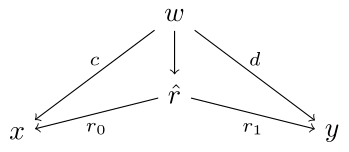commutes. We will write $r \colon c \nrightarrow d$ when $c$ is $r$-related to $d$.

While we can talk about relations internal to $any$ category, we cannot generally assemble them into another category. However, if we start with a regular category $E$, then there is a bicategory $\mathbf{Rel}(E)$ of relations internal to $E$. The objects are those of $E$. The arrows are the relations internal to $E$ with composition given by pullback: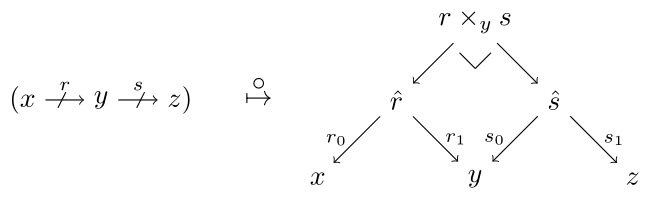Additionally, we have a unique 2-cell, written $r \leq s$, whenever $s \colon r_0 \nrightarrow r_1$. Diagrammatically, $r \leq s$ if there exists a commuting diagram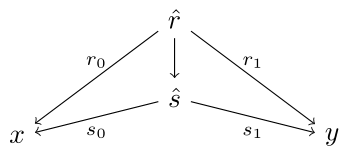### Internal ordered objects

We are quite used to the idea of having an order on a set. But what about an order on a category? This is captured by $\mathbf{Ord}(E),$ the bicategory of ordered objects and ideals in a regular category $E$.

The objects of $\mathbf{Ord}(E)$ are ordered objects in $E$. An ordered object is a pair $(x,r)$ consisting of an $E$-object $x$ and a reflexive and transitive relation $r : x \to x$ internal to $E$.

(Puzzle: $r$ is a monic of type $r \colon \hat{r} \to x \times x$. Both reflexivity and transitivity can be defined using morphisms. What are the domains and codomains? What properties should be satisfied?)

The arrows of $\mathbf{Ord}(E)$ are a sort of ‘order preserving relation’ called an ideal. Precisely, an ideal $f \colon (x,r) \to (y,s)$ between ordered objects is a relation $f \colon x \nrightarrow y$ such that given

• morphisms $a , a' , b , b'$ with a common domain $z$, and

• relations $r \colon a \nrightarrow a'$, $f \colon a' \nrightarrow b'$, and $s \colon b' \nrightarrow b$

then $f \colon a \nrightarrow b$.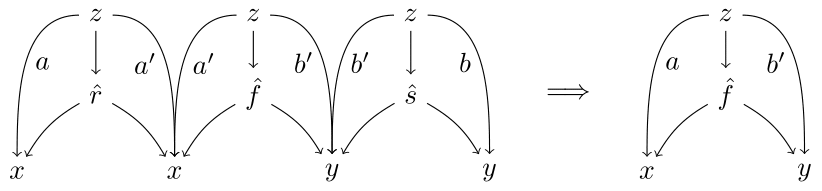In $\mathbf{Set}$, an ordered object is a preordered set and an ideal $f \colon (x,r) \to (y,s)$ is a directed subset of $x \times y$ with the property that if it contains $s$ and $s' \leq s$, then it contains $s'$.

There is at most a single 2-cell between parallel arrows in $\mathbf{Ord}(E)$. Given $f , g \colon (x,r) \to (y,s)$, write $f \leq g$ whenever $g \colon f_0 \nrightarrow f_1$.

## Cartesian Bicategories

Now that we know what bicategories we have the pleasure of working with, we can move forward with the theoretical aspects. As we work through the upcoming definitions, it is helpful to recall our motivating examples $\mathbf{Rel}(E)$ and $\mathbf{Ord}(E)$.

As mentioned above, in the early days of bicategory theory, mathematicians would study bicategories as $V$-enriched profunctor bicategories for some suitable $V$. A shrewd observation was made that when $V$ is Cartesian, a $V$-profunctor bicategory has several important commonalities with $\mathbf{Rel}(E)$ and $\mathbf{Ord}(E)$. Namely, there is the existence of a Cartesian product $\otimes$, plus for each object $x$, a diagonal arrow $\Delta \colon x \to x \otimes x$ and terminal object $\epsilon \colon x \to I$. With this insight, Carboni and Walters decided to take this structure as primitive.

To simplify coherence, we only look at locally posetal bicategories (i.e. $\mathbf{Pos}$-enriched categories). This renders 2-dimensional coherences redundant as all parallel 2-cells manifestly commute. This assumption also endows each hom-poset with 2-cells $\leq$ and, as we will see, local meets $\wedge$. For the remainder of this article, all bicategories will be locally posetal unless otherwise stated.

Definition. A locally posetal bicategory $B$ is Cartesian when equipped with

• a symmetric tensor product $B \otimes B \to B$,

• a cocommutative comonoid structure, $\Delta_x \colon x \to x \otimes x$, and $\epsilon_x \colon x \to I$, on every $B$-object $x$

such that

• every 1-arrow $r \colon x \to y$ is a lax comonoid homomorphism, i.e.

$\Delta_y r \leq ( r \otimes r ) \Delta_x \quad \text{and} \quad \epsilon_y r \leq \epsilon_x$

• for all objects $x$, both $\Delta_x$ and $\epsilon_x$ have right adjoints $\Delta^\ast_x$ and $\epsilon^\ast_x$.

Moreover, $(\Delta_x , \epsilon_x)$ is the only cocommutative comonoid structure on $x$ admitting right adjoints.

(Question: This definition contains a slight ambiguity in the authors use of the term “moreover”. Is the uniqueness property of the cocommutative comonoid structure an additional axiom or does it follow from the other axioms?)

If you’re not accustomed to thinking about adjoints internal to a general bicategory, place yourself for a moment in $\mathbf{Cat}$. Recall that adjoint functors are merely a pair of arrows (adjoint functors) together with a pair of 2-cells (unit and counit) obeying certain equations. But this sort of data can exist in any bicategory, not just $\mathbf{Cat}$. It is worth spending a minute to feel comfortable with this concept because, in what follows, adjoints play an important role.

Observe that the right adjoints $\Delta^\ast_x$ and $\epsilon^\ast_x$ turn $x$ into a commutative monoid object, hence a bimonoid. The (co)commutative (co)monoid structure on an object $x$ extend to a tensor product on $x \otimes x$ as seen in this string diagram: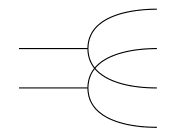Ultimately, we want to think of arrows in a Cartesian bicategory as generalized relations. What other considerations are required to do this? To answer this, it is helpful to first think about what a generalized function should be.

For the moment, let’s use our $\mathbf{Set}$ based intuition. For a relation to be a function, we ask that every element of the domain is related to an element of the codomain (entireness) and that the relationship is unique (determinism). How do we encode these requirements into this new, general situation? Again, let’s use intuition from relations in $\mathbf{Set}$. Let $r \nrightarrow x \times y$ be a relation and $r^\circ \nrightarrow y \times x$ be the relation defined by $r^{\circ} \colon y \nrightarrow x$ whenever $r \colon x \nrightarrow y$. To say that $r$ is entire is equivalent to saying that the composite relation $r^\circ r$ contains the identity relation on $x$ (puzzle). To say that $r$ is deterministic is to say that the composite relation $rr^\circ$ is contained by the identity (another puzzle). These two containments are concisely expressed by writing $1 \leq r^\circ r$ and $r r^\circ \leq 1$. Hence $r$ and $r^\circ$ form an adjoint pair! This leads us to the following definition.

Definition. An arrow of a Cartesian bicategory is a map when it has a right adjoint. Maps are closed under identity and composition. Hence, for any Cartesian bicategory $B$, there is the full subbicategory $\mathbf{Map}(B)$ whose arrows are the maps in $B$.

(Puzzle: There is an equivalences of categories $E \simeq \mathbf{Map}(\mathbf{Rel}(E))$ for a regular category $E$. What does this say for $E := \mathbf{Set}$?)

We can now state what appears as Theorem 1.6 of the paper. Recall that $(-)^\ast$ refers to the right adjoint.

Theorem. Let $B$ be a locally posetal bicategory. If $B$ is Cartesian, then

• $\mathbf{Map}(B)$ has finite bicategorical products $\otimes$,

• the hom-posets have finite meets $\wedge$ (i.e. categorical products) and the identity arrow in $B(I,I)$ is maximal (i.e. a terminal object), and

• bicategorical products and biterminal object in $\mathbf{Map}(B)$ may be chosen so that $r \otimes s = (p^\ast r p) \wedge (p s p^\ast)$,where $p$ denotes the appropriate projection.

Conversely, if the first two properties are satisfied and the third defines a tensor product, then $B$ is Cartesian.

This theorem gives a nice characterisation of Cartesian bicategories. The first two axioms are straightforward enough, but what is the significance of the above tensor product equation?

It’s actually quite painless when you break it down. Note, every bicategorical product $\otimes$ comes with projections $p$ and inclusions $p^\ast$. Now, let $r \colon w \to y$ and $s \colon x \to z$ which gives $r \otimes s \colon w \otimes x \to y \otimes z$. One canonical arrow of type $w \otimes x \to y \otimes z$ is $p^\ast r p$ which first projects to $w$, arrives at $y$ via $r$, which then includes into $y \otimes w$. The other arrow is similar, except we first project to $x$. The above theorem says that by combining these two arrows with a meet $\wedge$, the only available operation, we get our tensor product.

## Characterizing bicategories of internal relations

The next stage is to add to Cartesian bicategories the property that each object is a Frobenius monoid. In this section we will study such bicategories and see that Cartesian plus Frobenius provides a reasonable axiomatization of relations.

Recall that an object with monoid and comonoid structures is called a Frobenius monoid if the equation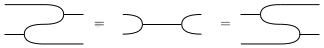holds. If you’re not familiar with this equation, it has an interesting history as outlined by Walters. Now, if every object in a Cartesian bicategory is a Frobenius monoid, we call it a bicategory of relations. This term is a bit overworked as it commonly refers to $\mathbf{Rel}(E)$. Therefore, we will be careful to call the latter a “bicategory of internal relations”.

Why are bicatgories of relations better than simply Cartesian bicategories? For one, they admit a compact closed structure! This appears as Theorem 2.4 in the paper.

Theorem. A bicategory of relations has a compact closed structure. Objects are self-dual via the unit

$\Delta \epsilon^\ast_x \colon I \to x \otimes x$

and counit

$\epsilon \Delta^\ast_x \colon x \otimes x \to I.$

Moreover, the dual $r^\circ$ of any arrow $r \colon x \to y$ satisfies

$(r \otimes id) \Delta \leq (1 \otimes r^\circ) \Delta r$

and

$\Delta^\ast (r \otimes id) \leq r \Delta^\ast (1 \otimes r^\circ).$

Or if you prefer string diagrams, the above inequalities are respectively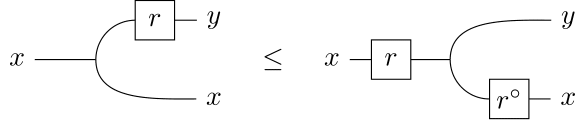and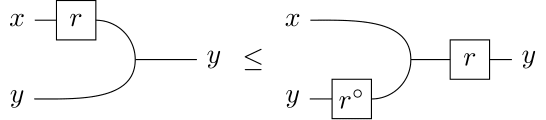Because a bicategory of relations is Cartesian, maps are still present. In fact, they have a very nice characterization here.

Lemma. In a bicategory of relations, an arrow $r$ is a map iff it is a (strict) comonoid homomorphism iff $r \dashv r^\circ$.

As one would hope, the adjoint of a map corresponds with the involution coming from the compact closed structure. The following corollary provides further evidence that maps are well-behaved.

Corollary. In a bicategory of relations:

• $f$ is a map implies $f^\circ = f^\ast$. In particular, multiplication is adjoint to comultiplication and the unit is adjoint to the counit.

• for maps $f$ and $g$, if $f \leq g$ then $f=g$.

But maps don’t merely behave in a nice way. They also contain a lot of the information about a Cartesian bicategory and, when working with bicategories of relations, the local information is quite fruitful too. This is made precise in the following corollary.

Corollary. Let $F \colon B \to D$ be a pseudofunctor between bicategories of relations. The following are equivalent:

• $F$ strictly preserves the Frobenius structure.

• The restriction $F \colon \mathbf{Map}(B) \to \mathbf{Map}(D)$ strictly preserves the comonoid structure.

• $F$ preserves local meets and $I$.

## Characterizing bicategories of internal relations

The entire point of the theory developed above is to be able to prove things about certain classes of bicategories. In this section, we provide a characterization theorem for bicategories of internal relations. Freyd had already given this characterization using allegories. However, he relied on a proof by contradiction whereas using bicategories of relations allows for a constructive proof.

A bicategory of relations is meant to generalize bicategories of internal relations. Given a bicategory of relations, we’d like to know when an arrow is “like an internal relation”.

Definition. An arrow $r \colon x \to y$ is a tabulation if there exists maps $f \colon z \to x$ and $g \colon z \to y$ such that $r = g f^\ast$ and $f^\ast f \wedge g^\ast g = 1_z$.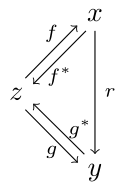This definition seems bizarre on its face, but it really is analogous to the jointly monic span-definition of an internal relation. That $r = g f^\ast$ is saying that $r$ is like a span $x \xleftarrow{f} z \xrightarrow{g} y$. The equation $f^\ast f \wedge g^\ast g = 1_z$ implies that this span is jointly monic (puzzle).

A bicategory of relations is called functionally complete if every arrow $r \colon x \to I$ has a tabulation $i \colon x_r \to x$ and $t \colon x_r \to I$. One can show that the existence of these tabulations together with compact closedness is sufficient to obtain a unique (up to isomorphism) tabulation for every arrow. We now provide the characterization, presented as Theorem 3.5.

Theorem. Let $B$ be a functionally complete bicategory of relations. Then:

• $\mathbf{Map}(B)$ is a regular category (all 2-arrows are trivial by an above corollary)

• There is a biequivalence of bicategories $\mathbf{Rel}(\mathbf{Map}(B)) \simeq B$ obtained by sending the relation $\langle f,g \rangle$ of $\mathbf{Rel}(\mathbf{Map}(B))$ to the arrow $g f^\circ$ of $B$.

So all functionally complete bicategories of relations are bicategories of internal relations. An interesting quesion is whether any regular category can be realized as $\mathbf{Map}(B)$ for some functionally complete bicategory of relations. Perhaps a knowledgeable passerby will gift us with an answer in the comments!

From this theorem, we can classify some important types of categories. For instance, bicategories of relations internal to a Heyting category are exactly the functionally complete bicategory of relations having all right Kan extensions. Bicategories of relations internal to an elementary topos are exactly the functionally complete bicategories of relations $B$ such that $B(x,-)$ is representable in $\mathbf{Map}(B)$ for all objects $x$.

## Characterizing ordered object bicategories

The goal of this section is to characterize the bicategory $\mathbf{Ord}(E)$ of ordered objects and ideals. We already introduced $\mathbf{Ord}(E)$ earlier, but that definition isn’t quite abstract enough for our purposes. An equivalent way of defining an ordered object in $E$ is as an $E$-object $x$ together with a relation $r$ on $x$ such that $1 \leq r$ and $r r \leq r$. Does this data look familiar? An ordered object in $E$ is simply a monad in $\mathbf{Rel}(E)$!

(Puzzle: What is a monad in a general bicategory? Hint: how are adjoints defined in a general bicategory?)

Quite a bit is known about monads, and we can now apply that knowledge to our study of $\mathbf{Ord}(E)$.

Recall that any monad in $\mathbf{Cat}$ gives rise to a category of adjunctions. The initial object of this category is the Kleisli category. Since the Kleisli category can be defined using a universal property, we can define a Kleisli object in any bicategory. In general, a Kleisli object for a monad $t \colon x \to x$ need not exist but when it does, it is defined as an arrow $k : x \to x_t$ plus a 2-arrow $\theta \colon k t \to k$ such that, given any arrow $f \colon x \to y$ and 2-arrow $\alpha \colon f t \to f$, there exists a unique arrow $h \colon x_t \to y$ such that $h k = f$. The pasting diagrams involved also commute: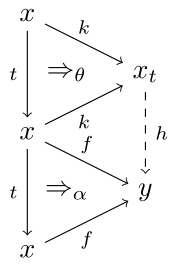As in the case of working inside $\mathbf{Cat}$, we would expect for $k$ to be on the left of an adjoint pair, and indeed it is. We get a right adjoint $k^\ast$ such that the composite $k^\ast k$ is our original monad $t$. The benefit of working in the locally posetal case is we also have that $k k^\ast = 1$. This realizes $t$ as an idempotent:

$t t = k^\ast k k^\ast k = k^\ast k = t.$

It follows that the Kleisli object construction is exactly an idempotent splitting of $t$! This means we can start with an exact category $E$ and construct $\mathbf{Ord}(E)$ by splitting the idempotents of $\mathbf{Rel}(E)$. With this in mind, we move on to the characterization, presented as Theorem 4.6.

Theorem. A bicategory $B$ is biequivalent to a bicategory $\mathbf{Ord}(E)$ if and only if

• $B$ is Cartesian,

• every monad in $B$ has a Kleisli object,

• for each object $x$, there is a monad on $x$ and a Frobenius monoid $x_0$ that is isomorphic to the monad’s Kleisli object,

• given a Frobenius monoid x and $f \colon x \to x$ with $f \leq 1$, $f$ splits.

## Final words

The authors go on to look closer at bicategories of relations inside Grothendieck topoi and abelian categories. Both of these are regular categories, and so fit into the picture we’ve just painted. However, each have additional properties and structure that compels further study.

Much of what we have done can be done in greater generality. For instance, we can drop the local posetal requirement. However, this would greatly complicate matters by requiring non-trivial coherence conditions.

Posted at February 19, 2018 7:50 PM UTC

TrackBack URL for this Entry:   https://golem.ph.utexas.edu/cgi-bin/MT-3.0/dxy-tb.fcgi/3020

### Re: Cartesian Bicategories

A very nice and comprehensive post! I have just a few comments/questions.

1. I presume that by “biproduct” you mean “bicategorical product”. Unfortunately it’s better not to do this, because “biproduct” also means something else. (Which is also related to cartesian bicategories: the third property in Theorem 1.6 is a sort of categorified version of this other notion of biproduct.)

2. Your definition of Frobenius monoid doesn’t seem right to me; at least, your additional axiom doesn’t coincide with the usual one (the last of the string diagram pictures here). Is it equivalent?

3. I didn’t know that Freyd used a proof by contradiction in characterizing allegories of internal relations. I would be surprised if that use were essential; have you looked at it? In particular, the theory of allegories is also exposited in chapter A3 of Sketches of an Elephant, and I don’t recall any use of excluded middle there; the Elephant is generally quite careful about noting such uses.

4. You asked whether any regular category can be realized as $\mathbf{Map}(B)$ for some functionally complete bicategory of relations $B$. Am I missing something? Can’t we just take $B = \mathbf{Rel}(E)$?

Posted by: Mike Shulman on February 20, 2018 5:42 PM | Permalink | Reply to this

### Re: Cartesian Bicategories

Thanks Mike!

1. Yes, I suppose this should be called a “2-product” - I don’t remember seeing that exact term anywhere, but nLab uses 2-limit, 2-pullback etc. (There’s a more general comment that the some of this paper’s terminology hasn’t stood the test of time very well, which doesn’t make it any easier to read. For example the paper uses “discrete object” for what we now call a Frobenius algebra. We spent quite a while in the seminar trying to understand why Carboni and Walters chose this term, without reaching much of a conclusion.)

2. I think the wrong image was included by mistake. This is the yanking/snake equation of a compact closed category. Sorry to everybody for confusion!

3. I’m going to leave this one for Daniel to answer ;)

4. It seems so. Daniel, is there a subtlety you had in mind here? (Can $\mathbf{Rel}(E)$ fail to be functionally complete?)

Posted by: Jules Hedges on February 20, 2018 10:31 PM | Permalink | Reply to this

### Re: Cartesian Bicategories

Hi Mike. Thanks for the comments.

1. As Julian already mentioned, you presume correctly. I’ll change the wording. This is a generalization of Fox who showed that including Cartesian categories into monoidal categories has a right adjoint (it was already known that a left adjoint exists). This right adjoint sends a monoidal category $C$ to the category of all $C$-coalgebras. Under this transformation, a generic tensor becomes a product.

2. Oops. Included the wrong image. Will fix.

3. I was taking the word of Carboni and Walters. On page 21 of Cartesian Bicategories, they claim Freyd used a proof by contradiction in his paper On canonizing category theory. I can’t seem to find this paper to verify their claim.

4. Looks like you are the knowledgeable passerby we were waiting for:) Taking $B=\mathbf{Rel}(E)$ is certainly the best candidate, but it wasn’t obvious to me that $\mathbf{Rel}(E)$ is functionally complete. Should it be obvious?

Posted by: Daniel Cicala on February 20, 2018 11:24 PM | Permalink | Reply to this

### Re: Cartesian Bicategories

1. I think the best explanation of the term “discrete” is that this condition ensures that the bicategory of maps is in fact locally discrete, i.e. equivalent to a 1-category. More specifically, if $y$ is Frobenius (i.e. “discrete”) then $\mathbf{Map}(B)(x,y)$ is equivalent to a discrete set. However, this depends on the prior assumption that $B$ is locally posetal; for a general cartesian bicategory, $y$ being Frobenius only implies that $\mathbf{Map}(B)(x,y)$ is a groupoid. The locally-posetal condition also has an “object-wise” version called being separable. These ideas are explored in the sequels of this paper which study non-locally-posetal cartesian bicategories: Cartesian bicategories II, Frobenius objects in cartesian bicategories, and Bicategories of spans as cartesian bicategories .

2. Looks much better now, thanks.

3. Wow, I’m not sure that I’ve seen before a reference to a “pamphlet”. I certainly have no idea how to go about finding it. But one could check Freyd and Scedrov’s book Categories, allegories to see whether Freyd was still using proof by contradiction by then. I hadn’t looked at the publication dates of these things before, but apparently Freyd’s pamphlet was “published” (?) in 1974, the Carboni-Walters paper was 13 years later in 1987, and then the Freyd-Scedrov book appeared 3 years after that in 1990. So it’s possible that Categories, allegories uses ideas adapted from Carboni-Walters (in particular, avoiding proof by contradiction) to replace Freyd’s original arguments in his pamphlet. Unfortunately, Categories, allegories also uses some rather idiosyncratic notation and terminology; the most readable treatment of allegory theory that I know of is chapter A3 of Sketches of an Elephant (though I can’t resist also mentioning section 6 of my own paper Exact completions and small sheaves).

4. Well, as you said in the post, the notion of tabulation is “analogous to the jointly monic span-definition of an internal relation.” So the jointly monic span that defines an internal relation ought to be a tabulation of that relation qua morphism in $\mathbf{Rel}(E)$.

Posted by: Mike Shulman on February 21, 2018 12:39 AM | Permalink | Reply to this

### Re: Cartesian Bicategories

Great stuff! I never read these papers by Carboni and Walters. I should have!

One nitpicky question:

We are quite used to the idea of having an order on a set. But what about an order on a category? This is captured $\mathbf{Ord}(E)$, the bicategory of ordered objects and ideals in a regular category $E$.

Why do you say “an order on a category”? It seems like instead you’re taking the concept of an order on a set and generalizing it to an order on an object in a category.

That’s what I’d call “internalization”: taking a concept defined in $Set$ and figuring out how to define it in any sufficiently similar category $E$.

If we can do it for $E = Cat$, internalization is sometimes called “categorification”.

Is $Cat$ regular? If so, what does an ordered object in $Cat$ amount to, exactly? That might be worth calling “an order on a category”.

Posted by: John Baez on February 21, 2018 7:12 AM | Permalink | Reply to this

### Re: Cartesian Bicategories

Yes, this should probably read “order on an object”, or “order in a category”. Sadly, https://ncatlab.org/nlab/show/regular+category directly says that Cat is not regular. (My first guess for what goes wrong would be that functors can be independently injective or surjective on objects and morphisms.)

From a casual Google, some people use “ordered category” for “locally posetal category”. I could attempt a from-scratch definition (say, a category $\mathcal{C}$ together with a functor to $\mathcal{C}$ from a poset on $\mathbf{Ob}(\mathcal{C})$), but I doubt it would be very interesting.

Posted by: Jules Hedges on February 21, 2018 9:19 AM | Permalink | Reply to this

### Re: Cartesian Bicategories

I don’t remember exactly what goes wrong with $Cat$ being regular, but probably it has to do with regular epis not being stable under pullback.

Fortunately, a category doesn’t need to be regular in order to define an internal order in it; it only has to have pullbacks. (Regularity is only needed if you want to compose ideals between such orders associatively.) So we can define ordered objects in $Cat$, which by the usual yoga of internalization are equivalently internal categories in $Pos$. An ordered object is just an internal category that happens to be a poset, so an ordered object in $Cat$ is a particular kind of double category, namely one which is “thin” (posetal) in one direction. This sort of double category coincidentally just showed up in another post.

Posted by: Mike Shulman on February 21, 2018 12:48 PM | Permalink | Reply to this

### Re: Cartesian Bicategories

Is there a diagram missing, right after this: “Diagrammatically, $r \leq s$ if there exists a commuting diagram” ?

Posted by: Simon Burton on February 23, 2018 10:21 PM | Permalink | Reply to this

### Re: Cartesian Bicategories

Yes, you’re right. Thanks for pointing that out. It’s fixed now.

Posted by: Daniel Cicala on February 24, 2018 6:27 PM | Permalink | Reply to this

### Re: Cartesian Bicategories

Hope some suggestions for references might be worth mentioning:

First, the series of posts on “Algebra of Processes” by Bob Walters (what a shame he passed away) that start with http://rfcwalters.blogspot.com/2014/04/on-algebra-of-processes.html These are listed here: http://rfcwalters.blogspot.com/search/label/category%20theory Moving on, http://www.tac.mta.ca/tac/volumes/19/6/19-06abs.html “Cartesian Bicats II” would seem relevant. In fact, if you visit http://tac.mta.ca/tac/ and do a simple page search on Walters, a number of papers might be of interest.

Posted by: Keith Harbaugh on March 1, 2018 11:45 PM | Permalink | Reply to this

### Re: Cartesian Bicategories

Oops, I should have included http://rfcwalters.blogspot.com/search/label/computing to the stuff at Walters’ blog. BTW, I wrote to him suggesting that he divide his blog into three blogs: one each on Mathematics, Computer Science, and Everything Else, but he said instead he would just use the labels plus cross references. I think by then his health was an issue.

Posted by: Keith Harbaugh on March 1, 2018 11:59 PM | Permalink | Reply to this

### Re: Cartesian Bicategories

Someone should have proved, or should prove, that for any regular category $E$ with finite coproducts, where finite limits distribute over finite coproducts, $\mathbf{Rel}(E)$ is a symmetric monoidal bicategory, with coproducts providing the monoidal structure. Kenny Courser and I proved this when $E$ is the category of finite-dimensional real vector spaces in our paper Coarse-graining open Markov processes. But now I’m already needing other examples!

Posted by: John Baez on March 6, 2018 4:21 PM | Permalink | Reply to this

### Re: Cartesian Bicategories

Proposition A3.2.11 in Sketches of an Elephant implies that a regular category $E$ is an extensive coherent category if and only if $Rel(E)$ has local finite unions and finite coproducts (which are then also finite products, so that $Rel(E)$ is both cartesian and cocartesian monoidal).

However, the category of finite-dimensional real vector spaces is not extensive or coherent, so this doesn’t apply. I’m actually a little surprised that it works in that case. What exactly do you mean by “finite limits distribute over finite coproducts”? There is a general notion of a kind of limit distributing over a kind of colimit, but for finite limits and coproducts it (at least the natural general form of it) implies pullback-stability of coproducts, which fails for vector spaces.

Posted by: Mike Shulman on March 6, 2018 6:16 PM | Permalink | Reply to this

### Re: Cartesian Bicategories

I’m pretty sure that what I need to work for vector spaces actually does work. But I could easily be making mistakes about the general abstract nonsense that underlies my specific concrete nonsense. Maybe it’s not pullbacks distributing over coproducts, but some other nice compatibility between pullbacks and coproducts, that lets direct sum of vector spaces give a monoidal structure on the bicategory of vector spaces, linear relations and inclusions of linear relations. I’ll think about it soon…. gotta teach now.

Posted by: John Baez on March 6, 2018 8:20 PM | Permalink | Reply to this

### Re: Cartesian Bicategories

Well, one thing is that I probably shouldn’t think of direct sums of vector spaces as coproducts — I should think of them as products! They’re both, but it’s their role as products here that matters.

How good does a category $E$ need to be for $\mathbf{Rel}(E)$ to become symmetric monoidal with the tensor product of objects being the product of objects in $E$?

Posted by: John Baez on March 7, 2018 4:17 PM | Permalink | Reply to this

### Re: Cartesian Bicategories

Haha, I should have thought of that. That’s true for any regular category. In fact it’s right up there in the post: the symmetric monoidal structure on a cartesian bicategory $B$ is the cartesian monoidal structure on $Map(B)$.

Posted by: Mike Shulman on March 8, 2018 12:19 AM | Permalink | Reply to this

### Re: Cartesian Bicategories

Okay, good. So the full story must be this: for any regular category $E$, there’s a symmetric monoidal double category $\mathbb{R}\mathbf{el}(E)$ with objects of $E$ as objects, relations in $E$ as horizontal 1-cells, morphisms in $E$ as vertical 1-morphisms, and some obvious 2-morphisms that I’m too lazy to explain now. The horizontal bicategory of this will be the symmetric monoidal bicategory $\mathbf{Rel}(E)$. Kenny and I did the case $E = FinVect_{\mathbb{R}}$.

Posted by: John Baez on March 8, 2018 2:00 AM | Permalink | Reply to this

### Re: Cartesian Bicategories

Yep. In fact, this full story follows from Theorem 14.2 of FB&MF applied to the subobject fibration of $C$. I don’t know whether this symmetric monoidal double category itself appears explicitly in print, but Example 5.8 of DTIMC mentions that the bicategory $Rel(E)$ can be obtained from this theorem.

Posted by: Mike Shulman on March 8, 2018 1:40 PM | Permalink | Reply to this

### Re: Cartesian Bicategories

Very nice. Think it’s needed to change b’ to b in the illustration at the definition of ideal.

Posted by: Jesús López on April 15, 2019 4:56 PM | Permalink | Reply to this

### Re: Cartesian Bicategories

Recently appeared:

• Brendan Fong, David I Spivak, Regular and relational categories: Revisiting ‘Cartesian bicategories I’, (arXiv:1909.00069)
Posted by: David Corfield on September 4, 2019 9:37 PM | Permalink | Reply to this

### Re: Cartesian Bicategories

A very helpful article on this subject is Evan Patterson’s 2017 article Knowledge Representation in Bicategories of Relations, which I stumbled on looking at the 2018 paper Graphical Regular Logic.

I have been looking for a few years for a good Category Theoretic model of RDF and OWL, the W3C Data and ontology set of standards falling under the Semantic Web and Linked Data projects (see Literature).

Patterson’s paper is one of the most helpful in this space, giving a olog view of RDF, and made this area very accessible to me, as I have been working with those technologies for over 15 years).

I have two questions regarding bicategories of relations:

1) Is there something like a Power Object in the bicategories of relations? In Milewski’s Category Theory for Programmers chapter 28.2 “Monoidal Categories” states that when it can be constructed, the right adjoint of a tensor product defines the internal hom of the monoidal category with V(A⊗B,C) ≅ V(A,[B,C]). If it were possible to define those I guess one would have something like a conditional if … then … construction.

2) Has there been any work on showing how a bicategory of relations, for which the internal language is either regular logic or coherent logic (when one adds ⊕ and ⊥) can be turned into a modal logic? Something along the lines of the process of taking adjunctions between slice categories as described by David Corfield in his article Modal Homotopy Type Theory?

Posted by: Henry Story on February 5, 2020 3:21 PM | Permalink | Reply to this

Post a New Comment